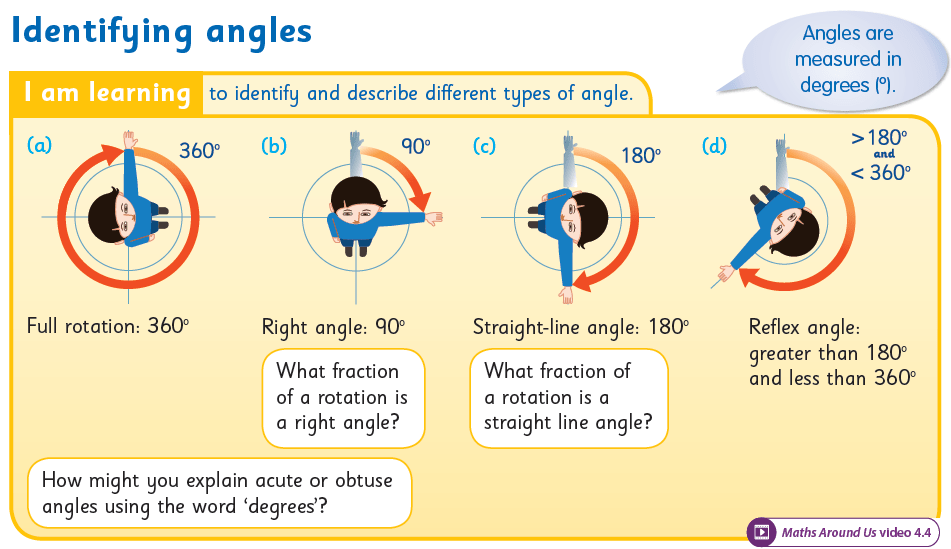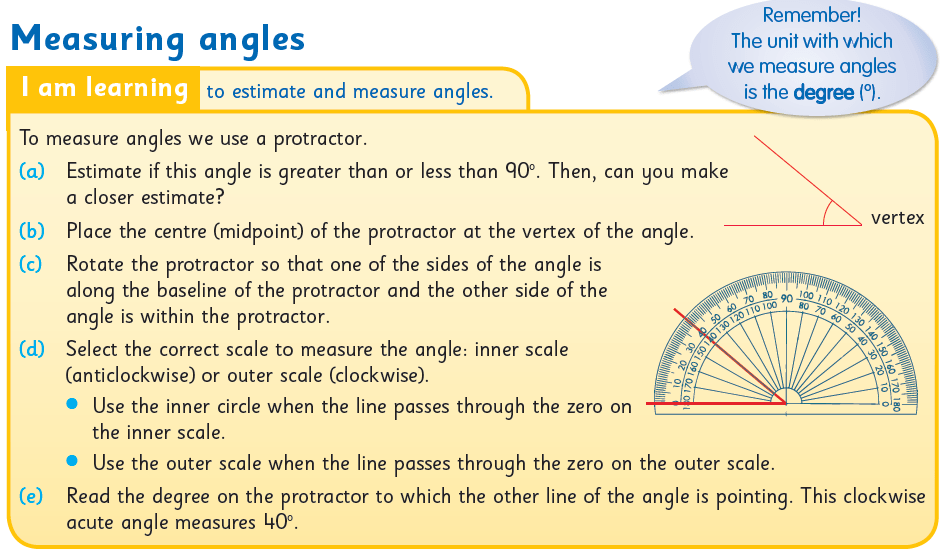# Digging Deeper into … Lines and Angles

## Digging Deeper into … Lines and Angles

Category : Uncategorized

For practical suggestions for families, and links to useful digital resources, to support children learning about the topic of place value, please check out the following post: Dear Family, your Operation Maths Guide to Lines and Angles

### Overview:

As can be seen from the overview table below, this topic is initially introduced to the children in 2nd class via “turns” and “square corners” and then develops with increasing complexity in 3rd- 6th classes.

 2nd 3rd 4th 5th 6th Lines vertical horizontal parallel perpendicular   oblique Angles Full, half, quarter turns, square corner Right angle   Greater/less than a right angle Acute angle   Obtuse angle Straight angle   Reflex angle Measuring and constructing in degrees Sum of angles in triangle  = 180° Sum of angles in quadrilateral  = 360°

As with every topic in Operation Maths, a CPA approach is also recommended for lines and angles:

• Concrete: allow sufficient time for the children to explore making turns, lines and angles with suitable concrete materials (e.g. the children themselves, lollipop sticks, straws, geo strips, construction materials, real-life examples from the school and home environment)
• Pictorial: activities where the focus is on drawing angles or lines on paper, MWBs etc
• Abstract: the final stage, where the focus is primarily on numbers,  digits  and or letters to represent variables eg given the measure of two angles in a triangle calculate the third angle.

### Lines:

Through exploration and activities, it is important that the children realise that:

• A line can be classified and identified according to its position and its relationship to another line.
• A single line can be horizontal, vertical or oblique but a single line cannot on its own be parallel or perpendicular; there must be two or more lines.
• Parallel lines do not all have to be the same length to be parallel.
• Parallel lines do not have to be horizontal or vertical, they can also be oblique.
• Perpendicular lines do not have to have a horizontal and vertical line, (again they can be oblique) but there must be at least one right angle where the two lines meet.

Lines can be drawn on the Operation Maths MWBs and then rotated to reinforce this point.
It is also worth noting that in maths, when we use the word “line”, it should be assumed that this is always straight; only if the word curved is given should it be assumed otherwise.

### Angles

In order for the children to recognise angles in terms of rotation, it is preferable initially, for the children to investigate the angles in their environment that are dynamic, (where the angle can be easily made bigger or smaller by increasing or decreasing the distance between the two lines) e.g. a door opening and closing, a scissors cutting paper, the angles made by the hands of a clock. The children can then proceed to examine static angles (where the angle is fixed) e.g. in 2-D shapes or 3D objects.Operation Maths, Pupils’ Book 3: on the digital book, click on the icon on the bottom right to access a “Ready to go” activity.

In second class (and revised at the start of third class), the concept of rotation of an angle is taught through the terms quarter-turn, half-turn and full turn. Ideally, this should be introduced concretely by getting the children themselves to do half-turns and quarter-turns, and to turn in clockwise and anticlockwise directions:

• In the classroom, the children start facing the board/front of room and make half/full/quarter turns to left/right as directed by the teacher.
• Repeat, but this time with different starting points
• Repeat, but this time after the teacher gives the directions the children must say where they will be facing, before they do the actual turn. The children could also record their predictions quickly on their Operation Maths MWBs

The children will be also be asked to identify 90º angles as square corners (2nd class) or right angles (3rd class). This will also be reinforced as part of the 2D shapes chapter. The children can be asked how they might decide if a corner/vertex of a shape is a square corner/right angle. Prior to the introduction of the protractor, something as simple as a corner torn from a piece of paper would suffice as an instrument with which to measure these angles.

The children should be enabled to classify angles according to the criteria appropriate to their class level (see table above). In particular, the ability to identify angles as acute (or less than a right angle), obtuse (or greater than a right angle) or reflex will greatly help the children, to later, estimate the measure of the angle in degrees, and to accurately measure and construct angles when they encounter this in 5th and 6th classes.Operation Maths, Pupils’ Book 5

It is important to constantly reinforce the children’s understanding of what an angle actually is, i.e. an amount of turn and that this can be represented by two adjoining lines, one showing the starting position, the other showing the point after the turn. Return to concrete examples if necessary; the children stretch out two arms in front and, leaving one arm in original position, they move other arm a certain amount (90 º, 180º etc). This could also be repeated using geostrips, connected at one end using a brass clip, so as to be able to move one of the ‘arms’. Such concrete experiences also link well to measuring using a protractor; the original arm is the ‘base’ line.

### Measuring and constructing angles (5th & 6th classes)Operation Maths, Pupils’ Book 5

Using degrees to describe angles is introduced in 5th class, which develops to include measuring and constructing angles using degrees. This necessitates the use of a protractor for the first time, which in itself can lead to difficulties. The child may be unsure where exactly to place the protractor; this can come from a lack of understanding of what an angle actually is and where the angle actually is. Also, a child can be uncertain of which scale to use to measure the size of the angle eg for an acute angle measuring 45º, the child writes down 135º.

To reduce the likelihood of this arising you can ask some/all of the following questions:

• What important tips would you give to a person about using a protractor?
• How do you know which scale to use on the protractor?
• What type of angle is this? How do you know? (To save time, they can write A, O or Re for Acute, Obtuse or Reflex).
• Estimate the measure of the angle to the nearest 10º. Is your estimate/measurement sensible? Why?
• How can you use what you know about acute and obtuse angles to check your measurement?

You can also watch some of the tutorial videos for using a protractor on the internet, such as the one below, for example. These videos can be a very visual way of demonstrating this skill. See also the list of digital resources for 5th and 6th classes on the following post: Dear Family, your Operation Maths Guide to Lines and Angles.

### Reasonableness of answer

Angles is another area where it is important for the child to check the reasonableness of the answer. First, the child needs to identify whether the angle is acute or obtuse. Then, if measuring an acute angle, the measurement must be less than 90º. If it isn’t, then the correct scale wasn’t used.

### Lines and Angles all around us

It is a given that lines and angles are all around us, although children may often be oblivious to the examples! Again, appropriate to each class level, the children should be encouraged to identify different types of lines and angles in their classroom, school and home. Enrich your own classroom space with lines and angles by labeling the line types in the room and the measure of angles of the open door. Make it personal by relating this topic to the children themselves and to the geometry in their names. Incorporate lines and angles into your visual art lessons (see also image below). Operation Maths 4 and 5 users can show the Maths Around Us video to their class, accessible on www.edcolearning.ie. For more ideas, check out this Lines & Angles board on Pinterest.Operation Maths, Pupils’ Book 4